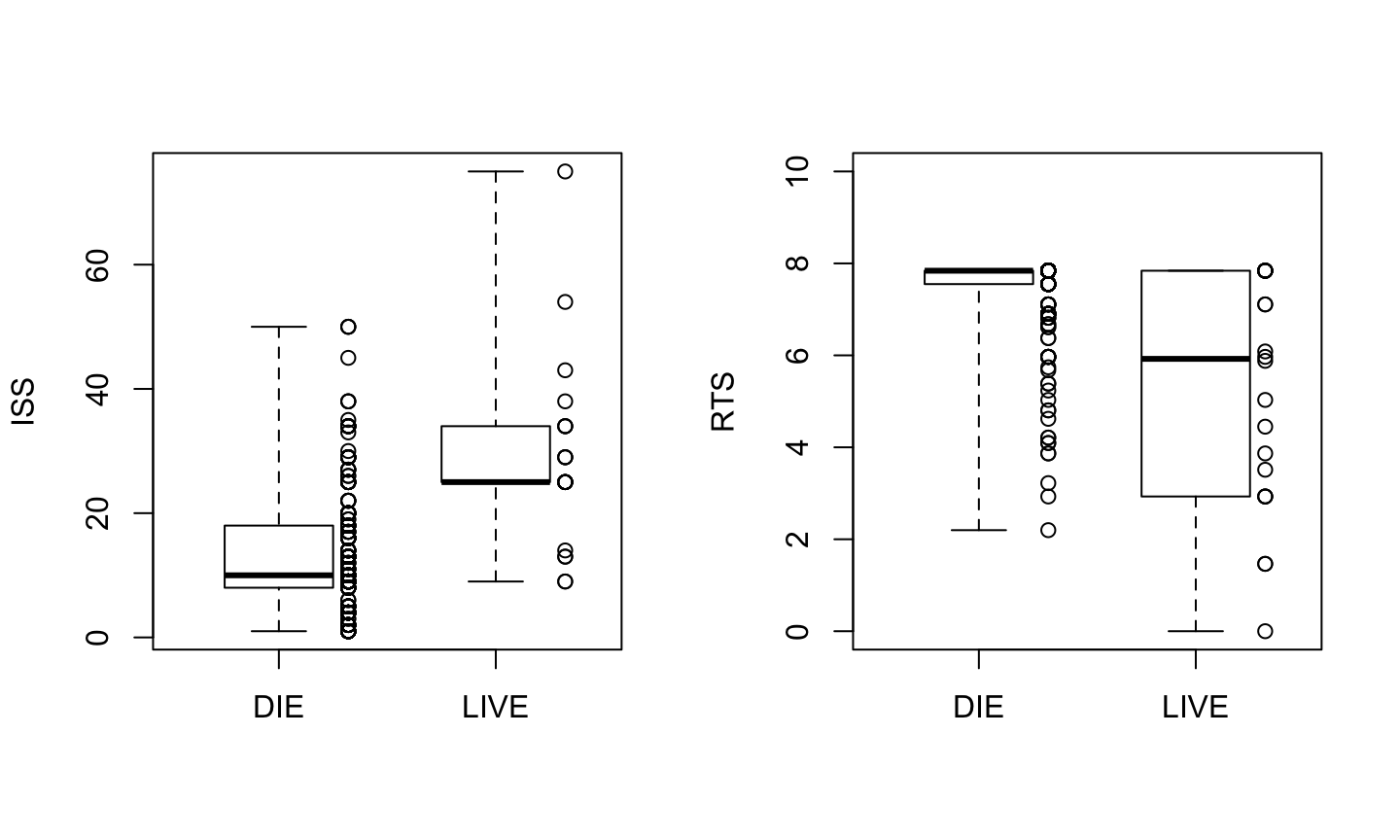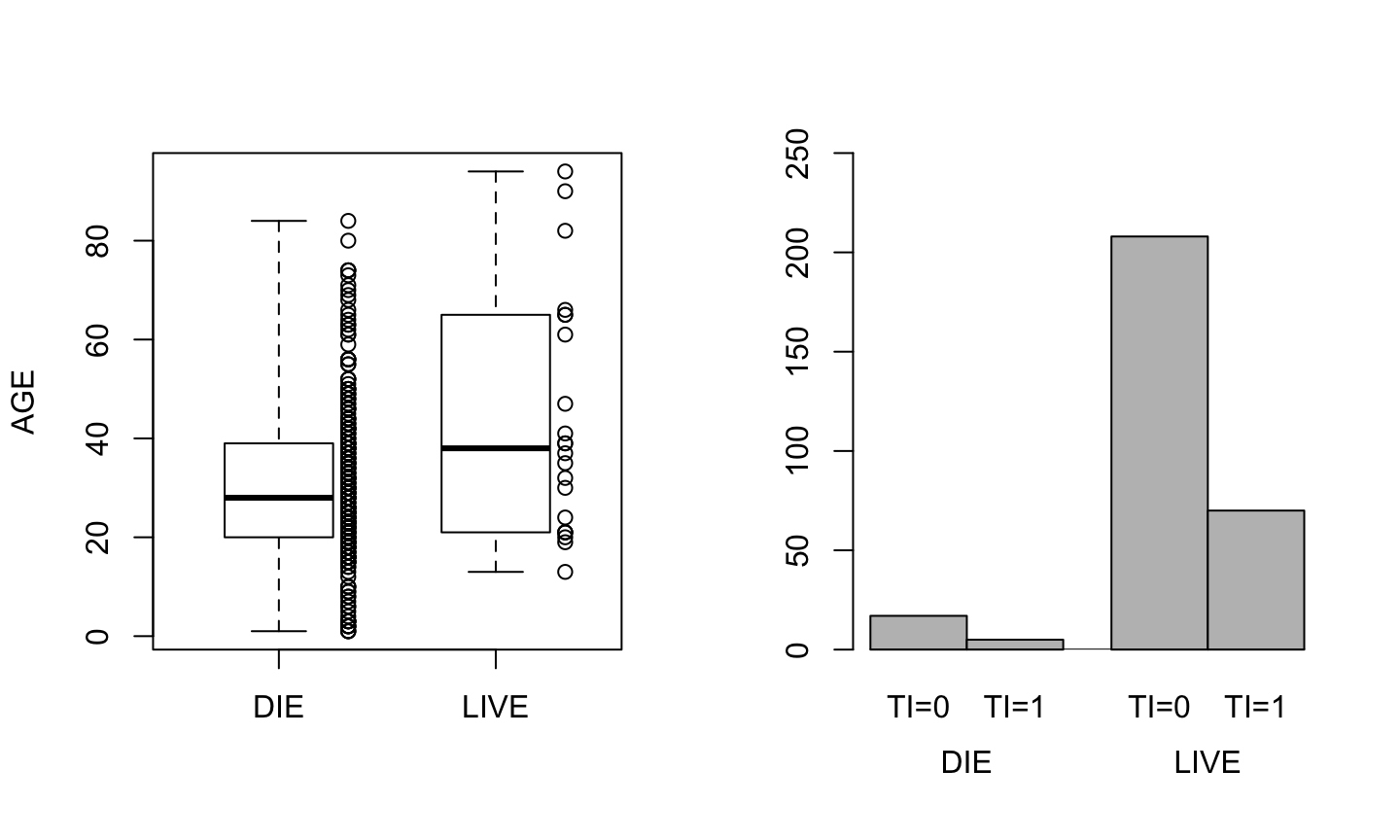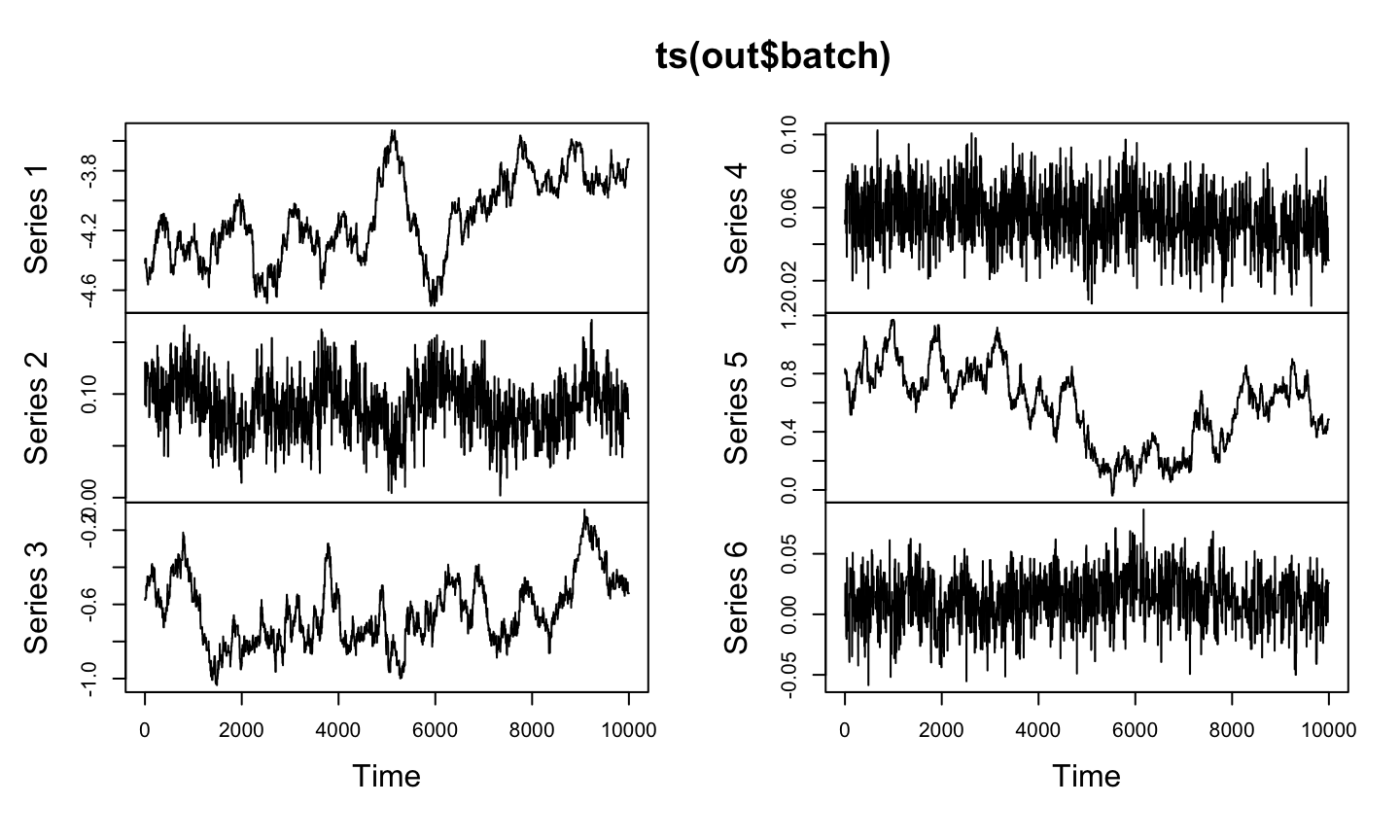## Agenda

• Motivating Example
• Binomial Regression
• Trauma Data Example
• Posterior Sampling with mcmc Package
• Exercise on O-Ring Data

## O-Ring Data

• On January 28, 1986, the space shuttle Challenger crashed after takeoff.
• Explosion was caused by field O-rings that failed due to the low atmospheric temperature at takeoff, 31 degrees Fahrenheit.
• Data on O-ring failure $$y$$ along with temperature at takeoff $$\check{x}$$ from the 23 pre-Challenger space shuttle launches. Each flight is viewed as an independent trial.
• Result of a trial is 1 if any field O-rings failed on the flight and 0 if all the O-rings functioned properly.
• We want to estimate the probability of O-ring failure as a function of temperature.

## O-Ring Data

• Data available here.
# Oring <- read.table("http://www.ics.uci.edu/~wjohnson/BIDA/Ch8/OringData.txt",
head(Oring)
##   Flight Failure Temperature
## 1     14       1          53
## 2      9       1          57
## 3     23       1          58
## 4     10       1          63
## 5      1       0          66
## 6      5       0          67

## O-Ring Data

• Let $$\theta_i$$ be the probability that any O-ring fails on launch $$i$$.
• Model the probability of O-ring failure as a simple function of temperature, i.e. use a straight line to model a function of the probabilities $\text{logit}(\theta_i) = \log \left[ \theta_i / (1-\theta_i) \right] = \beta_1 + \beta_2 \check{x}_i,$ where $$\check{x}_i$$ is the temperature corresponding to launch $$i$$.
• Define $x_i = \left[ \begin{matrix} 1 \\ \check{x}_i \end{matrix} \right] \text{ and } \beta = \left[ \begin{matrix} \beta_1 \\ \beta_2 \end{matrix} \right],$ then write the model as $$\text{logit}(\theta_i) = x_i ' \beta$$.

## Standardization

• Often convenient in regression problems to standardize continuous covariates so as to improve the performance of computational methods.
• Simplest standardization is to subtract the mean from any covariates.
• Writing $$\bar{x}$$ as the sample mean, O-ring model becomes $y_i | \theta_i \sim \text{Bin}(1, \theta_i), \quad \text{logit}(\theta_i) = \alpha + \beta_2 \left( \check{x}_i - \bar{x} \right).$
• For the O-ring data, standardization is helpful but not necessary.
• In more complicated problems, numerical procedures may be difficult to perform without standardization.
• Standardization can also affect the specification of prior information.

## Binomial Regression

• Consider data $$y_i$$, $$i = 1, \dots, n$$ with $y_i | \theta_i \stackrel{ind}{\sim} \text{Bin}(N_i, \theta_i).$
• Associated with each observation $$y_i$$ are $$r$$ fixed predictor variables $$x_{i1}, \dots, x_{ir}$$.
• Models use linear functions of the predictor variables but we need to take some function of the probabilities that transforms values between 0 and 1 into values on the real line.

## Binomial Regression

• A convenient class of functions that does this consists of the inverses of cumulative distribution functions.
• If $$F$$ is a cdf, its inverse is $$F^{-1}$$, then the general binomial regression model specifies $F^{-1} (\theta_i) = \sum_{j=1}^r \beta_j x_{ij} = x_i' \beta,$ where $$x_i' = \left( x_{i1}, \dots, x_{ir} \right)$$ and $$\beta = \left( \beta_1, \dots, \beta_r \right)'$$.
• Equivalently, $\theta_i = F(x_i' \beta).$
• Usually $$x_{i1}=1$$ so $$x_i' = \left( 1, x_{i2}, \dots, x_{ir} \right)$$ and $$\beta_1$$ is an intercept.

## Likelihood

• Likelihood function for independent binomial observations written as a vector $$y = (y_1, \dots, y_n)'$$ is \begin{aligned} L (\beta | y) & = \prod_{i=1}^n L (\beta | y_i) \\ & = \prod_{i=1}^n {N_i \choose y_i} \theta_i^{y_i} \left( 1 - \theta_i \right)^{N_i - y_i} \\ & = \prod_{i=1}^n {N_i \choose y_i} \left[ F (x_i' \beta ) \right]^{y_i} \left[ 1 - F (x_i' \beta ) \right]^{N_i - y_i} . \end{aligned}

## O-Ring Data MLE

model1 <- glm(Failure ~ Temperature, data = Oring,
family = binomial(), x = TRUE)
summary(model1)
##
## Call:
## glm(formula = Failure ~ Temperature, family = binomial(), data = Oring,
##     x = TRUE)
##
## Deviance Residuals:
##     Min       1Q   Median       3Q      Max
## -1.0611  -0.7613  -0.3783   0.4524   2.2175
##
## Coefficients:
##             Estimate Std. Error z value Pr(>|z|)
## (Intercept)  15.0429     7.3786   2.039   0.0415 *
## Temperature  -0.2322     0.1082  -2.145   0.0320 *
## ---
## Signif. codes:  0 '***' 0.001 '**' 0.01 '*' 0.05 '.' 0.1 ' ' 1
##
## (Dispersion parameter for binomial family taken to be 1)
##
##     Null deviance: 28.267  on 22  degrees of freedom
## Residual deviance: 20.315  on 21  degrees of freedom
## AIC: 24.315
##
## Number of Fisher Scoring iterations: 5

## Trauma Data

Dr. Turner Osler, a trauma surgeon and former head of the Burn Unit at the University of New Mexico Trauma Center, provided data on survival of patients admitted to the University of New Mexico Trauma Center between 1991 and 1994.

• The predictor variables are injury severity score (ISS), revised trauma score (RTS), AGE, and type of injury (TI). TI is either blunt ($$TI = 0$$), e.g., the result of a car crash, or penetrating ($$TI = 1$$), e.g., gunshot or stab wounds.
• ISS is an overall index of a patient's injuries based on the approximately 1,300 injuries cataloged in the Abbreviated Injury Scale. Takes on values from 0 for a patient with no injuries to 75 for a patient with severe injuries in three or more body areas.
• RTS is an index of physiologic status derived from the Glasgow Coma Scale. It is a weighted average of a number of measurements on an incoming patient such as systolic blood pressure and respiratory rate. Takes on values from 0 for a patient with no vital signs to 7.84 for a patient with normal vital signs.

## Trauma Data

• Data available here.
# trauma <- read.table("http://www.ics.uci.edu/~wjohnson/BIDA/Ch8/trauma300.txt,
head(traumadata)
##     ID death ISS TI    RTS AGE
## 1 2979     0   1  1 7.8408  25
## 2 1167     0   9  0 7.8408  63
## 3  116     0  29  0 2.9304  32
## 4 1118     0  10  0 7.8408  61
## 5 3126     0   2  1 7.8408  43
## 6 2173     0   9  0 7.8408  27

## Trauma Data## Trauma Data## Trauma Data

Dr. Osler proposed a logistic regression model to estimate the probability of a patient's death using an intercept; the predictors ISS, RTS, AGE, and TI; and an interaction between AGE and TI. AGE is viewed as a surrogate for a patient's physiologic reserve, i.e., their ability to withstand trauma.

The model $\text{logit}(\theta_i) = \beta_1 + \beta_2 ISS_i + \beta_3 RTS_i + \beta_4 AGE_i + \beta_5 TI_i + \beta_6 (AGE*TI)_i$ is similar to logistic models used by trauma centers throughout the United States.

Introduce $$x_{i6} = (AGE*TI)_i$$ and correct for the mean values of all the continuous predictor variables, then the model becomes $\text{logit}(\theta_i) = \alpha + \beta_2 x_{i2} + \beta_3 x_{i3} + \beta_4 x_{i4} + \beta_5 x_{i5} + \beta_6 x_{i6}.$

## Trauma Data

x.check <- cbind(ISS-mean(ISS), RTS-mean(RTS), AGE-mean(AGE), TI, AGE*TI-mean(AGE*TI))
colnames(x.check) <- c("x2", "x3", "x4", "x5", "x6")
head(x.check)
##          x2        x3    x4 x5        x6
## [1,] -13.28  0.553934 -6.41  1 17.633333
## [2,]  -5.28  0.553934 31.59  0 -7.366667
## [3,]  14.72 -4.356466  0.59  0 -7.366667
## [4,]  -4.28  0.553934 29.59  0 -7.366667
## [5,] -12.28  0.553934 11.59  1 35.633333
## [6,]  -5.28  0.553934 -4.41  0 -7.366667
model2 <- glm(death ~ x.check, family = binomial(), x = TRUE)

## Trauma Data

##
## Call:
## glm(formula = death ~ x.check, family = binomial(), x = TRUE)
##
## Deviance Residuals:
##     Min       1Q   Median       3Q      Max
## -2.5388  -0.2921  -0.1912  -0.1135   2.9019
##
## Coefficients:
##              Estimate Std. Error z value Pr(>|z|)
## (Intercept) -3.934673   0.596240  -6.599 4.14e-11 ***
## x.checkx2    0.082666   0.027508   3.005  0.00265 **
## x.checkx3   -0.552683   0.170824  -3.235  0.00121 **
## x.checkx4    0.053456   0.016382   3.263  0.00110 **
## x.checkx5    1.338003   1.333584   1.003  0.31571
## x.checkx6   -0.005013   0.031696  -0.158  0.87433
## ---
## Signif. codes:  0 '***' 0.001 '**' 0.01 '*' 0.05 '.' 0.1 ' ' 1
##
## (Dispersion parameter for binomial family taken to be 1)
##
##     Null deviance: 157.31  on 299  degrees of freedom
## Residual deviance: 100.03  on 294  degrees of freedom
## AIC: 112.03
##
## Number of Fisher Scoring iterations: 7

## Binomial Regression Analysis

• But this class isn’t about that frequentist analysis, we want a Bayesian analysis.
• For our Bayesian analysis we assume the same data model as the frequentist, i.e. we continue to use the logit link function.
• Bayesian analysis requires specification of a prior distribution.
• We assume the prior distribution of the parameters (the regression coefficients) makes them independent and identically normally distributed with mean 0 and variance $$\sigma^2 = 25$$.

## Unnormalized Posterior

• Suppose $$\eta = x'\beta$$ where $$x$$ is the design matrix.
• The log unnormalized posterior (log likelihood plus log prior) density for this model is $\sum_{i=1}^n y_i \log \left[ \frac{e^{\eta}}{1+e^{\eta}} \right] + \sum_{i=1}^n (N_i - y_i) \log \left[ \frac{1}{1+e^{\eta}} \right] + \frac{1}{2 \sigma^2} \sum_{j=1}^r \beta_j^2.$

## Unnormalized Posterior

• The log unnormalized posterior density calculated by the following R function.
x <- model2$x y <- model2$y
lupost <- function(beta, x, y) {
eta <- as.numeric(x %*% beta)
logp <- ifelse(eta < 0, eta - log1p(exp(eta)), - log1p(exp(- eta)))
logq <- ifelse(eta < 0, - log1p(exp(eta)), - eta - log1p(exp(- eta)))
logl <- sum(logp[y == 1]) + sum(logq[y == 0])
return(logl - sum(beta^2) / 50)
}

## Tricky Calculation

• The tricky calculation of the log likelihood avoids overflow and catastrophic cancellation in calculation of $$\log(p)$$ and $$\log(q)$$ where \begin{aligned} p & = \frac{e^{\eta}}{1+e^{\eta}} = \frac{1}{1+e^{-\eta}} \\ q & = \frac{1}{1+e^{\eta}} = \frac{e^{-\eta}}{1+e^{-\eta}}. \end{aligned}
• So taking logs gives \begin{aligned} \log (p) & = \eta - \log (1+e^{\eta}) = - \log (1+e^{-\eta}) \\ \log (q) & = - \log (1+e^{\eta}) = -\eta - \log (1+e^{-\eta}). \end{aligned}
• To avoid overflow, we always chose the case where the argument of $$\exp$$ is negative.

## Tricky Calculation

• We have also avoided catastrophic cancellation when $$| \eta |$$ is large.
• If $$\eta$$ is large and positive, then \begin{aligned} p & \approx 1 \\ q & \approx 0 \\ \log (p) & \approx - e^{-\eta} \\ \log (q) & \approx -\eta - e^{-\eta} \end{aligned} and our use of the R function log1p, which calculates the function correctly for small $$x$$ avoids all problems.
• Case where $$\eta$$ is large and negative is similar.

## Posterior Simulations

• Use mcmc package and metrop function to obtain posterior simulations.
• Following code runs the Metropolis algorithm to simulate the posterior.
library(mcmc)
set.seed(1)
beta.init <- as.numeric(coefficients(model2))
out <- metrop(lupost, beta.init, 1e3, x = x, y = y)
names(out)
##   "accept"       "batch"        "initial"      "final"
##   "accept.batch" "initial.seed" "final.seed"   "time"
##   "lud"          "nbatch"       "blen"         "nspac"
##  "scale"        "debug"
out$accept ##  0 ## Posterior Simulations • Acceptance rate is 0! We need to adjust the scale input. • Generally accepted that an acceptance rate of about 20% is right, although this recommendation is based on the asymptotic analysis of a toy problem (simulating a multivariate normal distribution) for which one would never use MCMC and is very unrepresentative of difficult MCMC applications. • The 20% magic number must be considered like other rules of thumb. That is, there are examples where they do fail, but it is something simple to tell beginners that works all right for many problems. ## Posterior Simulations out <- metrop(out, scale=0.1, x = x, y = y) out$accept
##  0.01
out <- metrop(out, scale=0.03, x = x, y = y)
out$accept ##  0.223 • Here the first argument to each instance of the metrop function is the output of a previous invocation. The Markov chain continues where the previous run stopped, doing just what it would have done if it had kept going, the initial state and random seed being the final state and random seed of the previous invocation. Everything stays the same except for the arguments supplied (here scale). ## Posterior Simulations • Argument scale controls the size of the Metropolis normal random walk proposal. • Default is scale = 1. • Big steps give lower acceptance rates. Small steps give higher. We want something about 20%. • Also possible to make scale a vector or a matrix. • Because each run starts where the last one stopped (when the first argument to metrop is the output of the previous invocation), each run serves as “burn-in” for its successor. ## Diagnostics • That does it for the acceptance rate. So let’s do a longer run and look at the results. out <- metrop(out, nbatch = 1e4, x = x, y = y) out$accept
##  0.1994
out$time ## user system elapsed ## 1.224 0.058 1.295 ## Diagnostics plot(ts(out$batch))## Diagnostics

acf(out\$batch[,1:3])